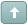Error

 Previous Topic Next Topic
 KTP2 #1 Posted : Wednesday, November 12, 2008 4:36:54 PM(UTC)Rank: Advanced MemberGroups: Registered, Registered Users, SubscribersJoined: 2/2/2007(UTC)Posts: 367Was thanked: 1 time(s) in 1 post(s) The function, system, and indicator introduced in the articles "Refining the Hilbert Indicator", by John Ehlers, and "Optimizing with Hilbert Indicators", by Roger Darley, which appear in the November 2000 TASC issue, can be created in MetaStock 7.0 or higher with the use of the new MetaStock External Function (MSX) DLL Interface. The C++ code and MetaStock formula code is shown below: MSX DLL C++ Code```// Main Calculation loop (begins after the fifth valid bar) for( l_iCurrentBar = a_iFirstValid + 5; l_iCurrentBar <= a_iLastValid;l_iCurrentBar++ ) { // Set Previous Bar counter l_iPreviousBar = l_iCurrentBar - 1; // Calculate Smoother and Detrender values l_pdSmoother[l_iCurrentBar] = (4 * a_pfPrice[l_iCurrentBar] + 3 * a_pfPrice[l_iPreviousBar] + 2 * a_pfPrice[l_iCurrentBar - 2] + a_pfPrice[l_iCurrentBar - 3])/10; l_pdDetrender[l_iCurrentBar] = (.25 * l_pdSmoother[l_iCurrentBar] + .75 * l_pdSmoother[l_iCurrentBar - 2] - .75 * l_pdSmoother[l_iCurrentBar - 4] - .25 * l_pdSmoother[l_iCurrentBar - 6])* double(.046f * a_pfPeriod[l_iPreviousBar] + .332f); // Calculate InPhase and Quadrature components l_pd_Q1[l_iCurrentBar] = (.25 * l_pdDetrender[l_iCurrentBar] + .75 * l_pdDetrender[l_iCurrentBar - 2] - .75 * l_pdDetrender[l_iCurrentBar - 4] - .25 * l_pdDetrender[l_iCurrentBar - 6]) * double(.046f * a_pfPeriod[l_iPreviousBar] + .332f); l_pd_I1[l_iCurrentBar] = l_pdDetrender[l_iCurrentBar - 3]; // Advance the phase of l_pd_I1 and l_pd_Q1 by 90 degrees l_pd_jI[l_iCurrentBar] = .25 * l_pd_I1[l_iCurrentBar] + .75 * l_pd_I1[l_iCurrentBar - 2] - .75 * l_pd_I1[l_iCurrentBar - 4] - .25 * l_pd_I1[l_iCurrentBar - 6]; l_pd_jQ[l_iCurrentBar] = .25 * l_pd_Q1[l_iCurrentBar] + .75 * l_pd_Q1[l_iCurrentBar - 2] - .75 * l_pd_Q1[l_iCurrentBar - 4] -.25 * l_pd_Q1[l_iCurrentBar - 6]; // Phasor addition to equalize amplitude due to quadrature // calculations (and 3 bar averaging) l_pd_I2[l_iCurrentBar] = l_pd_I1[l_iCurrentBar] - l_pd_jQ[l_iCurrentBar]; l_pd_Q2[l_iCurrentBar] = l_pd_Q1[l_iCurrentBar] + l_pd_jI[l_iCurrentBar]; // Smooth I and Q components before applying the discriminator l_pd_I2[l_iCurrentBar] = ForceFloatRange (.15 * l_pd_I2[l_iCurrentBar] + .85 * l_pd_I2[l_iPreviousBar]); l_pd_Q2[l_iCurrentBar] = ForceFloatRange (.15 * l_pd_Q2[l_iCurrentBar] + .85 * l_pd_Q2[l_iPreviousBar]); // Homodyne Discriminator; Complex Conjugate Multiply l_pd_X1[l_iCurrentBar] = l_pd_I2[l_iCurrentBar] * l_pd_I2[l_iPreviousBar]; l_pd_X2[l_iCurrentBar] = l_pd_I2[l_iCurrentBar] * l_pd_Q2[l_iPreviousBar]; l_pd_Y1[l_iCurrentBar] = l_pd_Q2[l_iCurrentBar] * l_pd_Q2[l_iPreviousBar]; l_pd_Y2[l_iCurrentBar] = l_pd_Q2[l_iCurrentBar] * l_pd_I2[l_iPreviousBar]; l_pd_Re[l_iCurrentBar] = l_pd_X1[l_iCurrentBar] + l_pd_Y1[l_iCurrentBar]; l_pd_Im[l_iCurrentBar] = l_pd_X2[l_iCurrentBar] - l_pd_Y2[l_iCurrentBar]; // Smooth to remove any undesired cross products l_pd_Re[l_iCurrentBar] = ForceFloatRange (.2 * l_pd_Re[l_iCurrentBar] + .8 * l_pd_Re[l_iPreviousBar]); l_pd_Im[l_iCurrentBar] = ForceFloatRange (.2 * l_pd_Im[l_iCurrentBar] + .8 * l_pd_Im[l_iPreviousBar]); // Compute Cycle Period if (l_pd_Im[l_iCurrentBar] != 0 && l_pd_Re[l_iCurrentBar] != 0) a_pfPeriod[l_iCurrentBar] = float (ForceFloatRange (360 / (atan(l_pd_Im[l_iCurrentBar] / l_pd_Re[l_iCurrentBar] ) * (180.0 / PI)))); if (a_pfPeriod[l_iCurrentBar] > 1.5f *a_pfPeriod[l_iPreviousBar]) a_pfPeriod[l_iCurrentBar] = 1.5f * a_pfPeriod[l_iPreviousBar]; if (a_pfPeriod[l_iCurrentBar] < .67f *a_pfPeriod[l_iPreviousBar]) a_pfPeriod[l_iCurrentBar] = .67f * a_pfPeriod[l_iPreviousBar]; if (a_pfPeriod[l_iCurrentBar] < 6 ) a_pfPeriod[l_iCurrentBar] = 6; if ( a_pfPeriod[l_iCurrentBar] > 50 ) a_pfPeriod[l_iCurrentBar] = 50; a_pfPeriod[l_iCurrentBar] = .2f * a_pfPeriod[l_iCurrentBar] + .8f * a_pfPeriod[l_iPreviousBar]; } ``` MetaStock Code Hilbert Channel Breakout Indicator entryk:=Input("Entry K", 0, 1, 0);entryval:=Input("Entry val", 0, 100, 0);exitk:= Input("Exit K", 0, 4.5, 0);exitval:=Input("Entry val", 0, 100, 0); ExtFml( "EnhancedHilbert.ChannelHigh", (HIGH+LOW)/2, entryk, entryval);ExtFml( "EnhancedHilbert.ChannelLow", (HIGH+LOW)/2, exitk, exitval) Hilbert Channel Breakout Signal Enter Long:H > ExtFml( "EnhancedHilbert.ChannelHigh",(HIGH+LOW)/2, 0, 15) Enter Short:L < ExtFml( "EnhancedHilbert.ChannelLow",(HIGH+LOW)/2, 0, 15) Click the links below to download the sample source code.Wanna join the discussion?! Login to your Discussions forum accountor Register a new forum account.Users browsing this topic
Forum Jump
You cannot post new topics in this forum.
You cannot reply to topics in this forum.
You cannot delete your posts in this forum.
You cannot edit your posts in this forum.
You cannot create polls in this forum.
You cannot vote in polls in this forum.